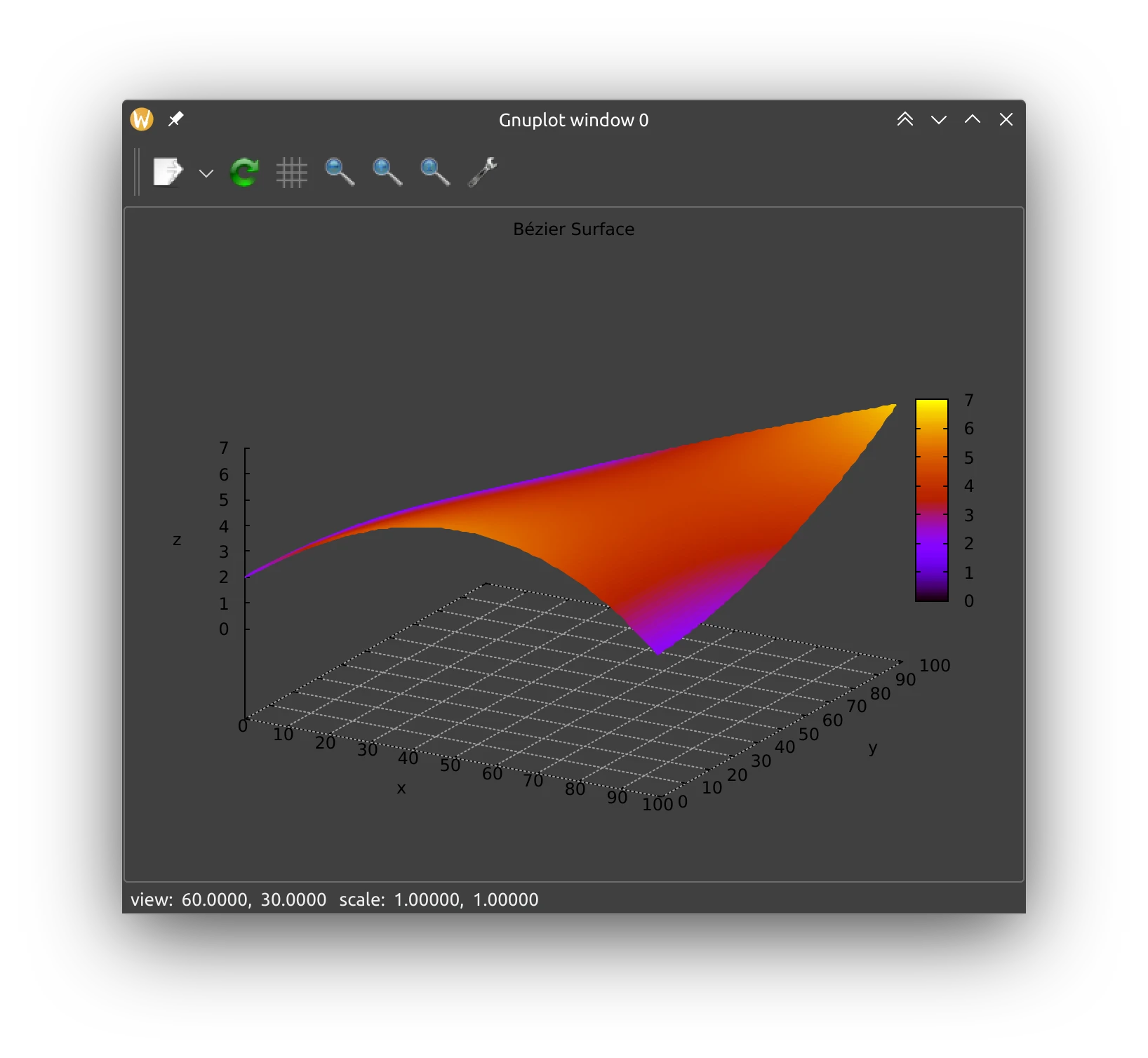# Isogeometric Analysis: Bézier Curves and Surfaces in Rust## Introduction

I recently implemented a demo of Isogeometric Analysis in Octave and then, just for fun, in TypeScript. Here are a few articles on the topic.

After writing the algorithms in TypeScript, I wondered what improvement I could get by using a compiled language. My first choice would be C++, but I though that it could be interesting to learn a bit of Rust. At the moment, I only implemented the basic linear algebra structures, but this is sufficient to add an implementation of Bézier curves and surfaces.

The implementation can be found in this crate: https://crates.io/crates/isogeometric_analysis. Docs for the crate can be found here: https://docs.rs/crate/isogeometric_analysis/latest.

## Bézier Curves

Creating a Bézier curve with the crate requires only to provide the control points in 2D or 3D space. With the following code I can evaluate the curve in equally spaced points in the $[0, 1]$ range:

use isogeometric_analysis::core::p2;
use isogeometric_analysis::bezier::{BezierCurve, BezierCurveDemo1};
use isogeometric_analysis::core::Evaluator;
let cpoints = BezierCurveDemo1::create().p;
let bez = BezierCurve::create(cpoints);
let (xpoints, ypoints) = Evaluator::<1, 2, 10000>::evaluate_parametric_range1d(&bez, &0f64, &1f64);

The same could be done with my TypeScript implementation with:

let bezier = new BezierCurve(exampleCurve2D1);
let output = new Point(0, 0);
let points = []
for (let i = 0; i < 10000; i++) {
let xi = i/10000;
points.push(bezier.evaluate_fill(xi, output));
}

The result is that evaluating $10^4$ points in this Bézier curve with the Rust implementation took $~1.5$ milliseconds, while the TypeScript implementation took $~13$ milliseconds on the JS engine provided by nodejs.

Please note that the above values are only approximate. The implementations are not optimized in any way and I am not an experienced Rust programmer.

By using the gnuplot crate I could also render the resulting curve:

## Bézier Surfaces

The Rust implementation also includes API to compute Bézier surfaces in the 3D space.

let bez = BezierSurf { data: cpoints };
let before = Instant::now();
let r = RealRange { a: 0f64, b: 1f64 };
let (_xpoints, ypoints) = Evaluator::<2, 3, 100>::evaluate_parametric_range2d(&bez, &r, &r);
let (_xvalues, _yvalues, zvalues) = Evaluator::<2, 3, 0>::split_coords(0, &ypoints, 1, &ypoints, 2, &ypoints);

gives this result:

Bye! 😉